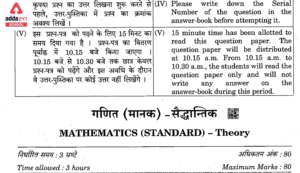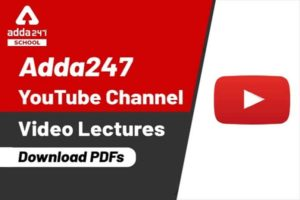Online Tution   »   Class 10   »   Algebra Formulas

# Algebra formulas PDF for SSC CGL and Class 10

## Algebra Formula

The Algebra formula is part of Class 10 in India. One of the most crucial areas of mathematics is algebra. Numerous disciplines, including quadratic equations, polynomials, coordinate geometry, calculus, trigonometry, probability, and others, can be solved using algebraic formulas. In Algebra formulas, we used numbers along with letters together. The most common letters used in algebraic equations and problems are X, Y, A, and B. These Algebra formulas enable us to quickly and efficiently tackle time-consuming algebraic problems. Here, we include all significant Algebra formulas together with their solutions, so that students can access them all in one place.

## Algebra Formulas

Algebra formulas are basically algebra equations formed by algebraic and mathematical phrases and symbols. These algebraic formulas contain an unknown variable x which can be generated while simplifying an equation. These algebraic equations solve complicated algebraic computations in an easy way.
For Algebra formulas example,

(a+b)³ =a³+ 3a²b+3ab²+b³
In the above Algebra formulas, both sides are individually an algebraic equation. Where ( a³ + 3a²b+3ab²+b³ ) is the simplified expression of (a+b)³ .

## Algebra formulas Square for Class 10

Here are some Algebra formulas involving squares.
• a²– b² = (a – b)(a + b)
• (a + b)²=  a²+ 2ab + b²
• a²+ b²= (a + b)²– 2ab
• (a – b)² = a²– 2ab+ b²
• (a + b + c)² = a² + b² + c²+ 2ab + 2bc + 2ca
• (a – b – c)² = a²+ b²+ c²– 2ab + 2bc – 2ca

## Algebra formulas Cube for SSC CGL

Here are some Algebra formulas involving cubes.

• (a + b)³ = a³+ 3a²b + 3ab²+ b³
•  (a + b)³ = a³ + b³ + 3ab(a + b)
• (a – b)³= a³ – 3a²b + 3ab² – b³
• (a – b)³= a³ – b³ – 3ab(a – b)
• a³ – b³ = (a – b)(a²+ ab + b²)
• a³ + b³ = (a + b)(a²– ab + b²)

Some more Algebra formulas are –
• (a + b)⁴= a⁴+ 4a³b + 6a²b² + 4ab³ + b²
• (a – b)⁴= a4 – 4a³b + 6a²b² – 4ab³+ b⁴
• a⁴ – b⁴= (a – b)(a + b)(a² + b²)
• a⁵ – b⁵= (a – b)(a⁴ + a³b + a²b² + ab³+ b⁴)

## Algebra formulas- Natural Numbers

Algebra formulas for Natural Numbers Except for 0 and negative numbers , the rest of the numbers [ 2 to infinity] in the number system that humans can count are known as Natural Numbrrs. Some algebraic formulas are applied when performing operations on natural numbers. They are.
Consider n to be a natural number.

1. (an – bn )= (a – b)(an-1 + an-2b+…+ bn-2a + bn-1)
2. ( an + bn)= (a + b)(an-1 – an-2b +…+ bn-2a – bn-1) [ where n is even  , (n = k + 1)  ]
3. (an + bn )= (a + b)(an-1 – an-2b +an-3b2…- bn-2a + bn-1) [ where n is odd , (n = 2k + 1)  ]

## Algebra formulas- Laws of Exponents

In Algebra formulas An Exponent or power is used to demonstrate the repeated multiplication of a number. Such as 3×3×3×3 can be written as 3⁴ where 4 is the exponent of 3.   In general, exponents or powers indicate how many times a number can be multiplied. There are various rules to operate an exponent for addition, subtraction, and multiplication which are easily solved by algebraic formulas.

## Algebra formulas- Quadric equations

Algebra formulas for Quadric equations are one of the most important topics in the syllabus of Class 9 and 10 . To find the root of the given  quadric equations we used the following Algebra formulas

If ax²+bx+c =0 is a quadric equation ,then

From the above formula we can conclude that , If the roots of the quadric equation are α and β
1. The equation will be (x − α)(x − β) = 0,
2.  The value of (α + β ) = (-b / a) and α × β = (c / a).

## Algebra formulas For irrational Numbers (SSC CGL)

The Algebra formulas used to solve equations based on Irrational Numbers are following

1. √ab = √a √b
2. √a/b =√a / √b
3. ( √a +√b )  ( √a – √b ) = a-b
4. ( √a +√b )²= a + 2 √ab + b
5. ( a +√b )( a -√b )= a² – b

## Algebra formulas List

Here a list of all important Algebra formulas is provided. Students must go through the list to solve difficult algebraic equations very quickly.

### Important Algebra Formulas

1 a²– b² = (a – b)(a + b)
2 (a + b)²=  a²+ 2ab + b²
3 a²+ b²= (a + b)²– 2ab
4 (a – b)² = a²– 2ab+ b²
5 (a + b + c)² = a² + b² + c²+ 2ab + 2bc + 2ca
6 (a – b – c)² = a²+ b²+ c²– 2ab + 2bc – 2ca
7 (a + b)³ = a³+ 3a²b + 3ab²+ b³
8 (a + b)³ = a³ + b³ + 3ab(a + b)
9 (a – b)³= a³ – 3a²b + 3ab² – b³
10 (a – b)³= a³ – b³ – 3ab(a – b)
11 a³ – b³ = (a – b)(a²+ ab + b²)
12 a³ + b³ = (a + b)(a²– ab + b²)
13 (a + b)⁴= a⁴+ 4a³b + 6a²b² + 4ab³ + b²
14 (a – b)⁴= a4 – 4a³b + 6a²b² – 4ab³+ b⁴
15 a⁴ – b⁴= (a – b)(a + b)(a² + b²)
16 a⁵ – b⁵= (a – b)(a⁴ + a³b + a²b² + ab³+ b⁴)

## Algebra Formulas PDF

Check Out The Algebra Formulas PDF for Class 10 Students. Click Here- Algebra formulas

## Algebra formulas with examples (solved)

Example 1 : Find the value 20²- 15²
Solution: To solve the  equation ,the formula we use is
a² -b² = (a+b) (a-b)
= (20+15)(20-15)
= 35 × 5

Example 2 : (x-y) =2 and x²+ y² =20 then find the value of x  and y [ where x,y >0 ]
Solution: Here , x²+ y² =20
(x-y)² +2xy =20
Or, (2)²+ 2xy =20
Or, 2xy = 20-4  = 16
Or,xy = 8
Now, (x+y)²= (x-y)² +4xy = (2)² +4.8 =36
Or,x+y = ± 6
So, x+y = 6 [x,y >0 ] ….(1)
We also get , x- y =2 ……(2)
Solving two equations we get ,
x = 4 and y = 2 (Answer)

Example 3 : Divide( a³ + b³ + c³ – 3abc )by( a+b+c )and the quotient.Determine the magnitude.

Solution: a+b³ + c3 – 3abc

=(a+b+c)(a²+b²+c2-ab-ac-bc)

Determinant quotient =
[(a+b+c)(a²+b²+c²-ab-ac-bc)] ÷ (a+b+c)

= a²+b²+c²-ab-be-ca.

Magnitude of quotient is  2. (Answer)

Example 4
Find their successive product (x + y), (x – y),  (x² + y²).

Solution :Determinant serial product =
(x + y) (x – y) (x² + y²)
= (x²-y²)(x² + y²)
= (x²)² – (y²)²
= x⁴ – y ⁴.( Answer)

## Algebra formulas- Questions

1. If x+y = 3 and xy = 2, what is the value of (x – y) ² ?
2. If a+b = 8 and ab = 15, what will be the values ​​of a and b?
3. If a+b = 5 and ab = 6, Find the value of a² – b² ?
4. If x = 29 and y = 14, what is the value of (4x² + 9y²+ 12xy )?

Related Post:

## Algebra formulas: FAQs

Q, What are formula used to solve algebra problems ?

Mainalgebra  formulas which are widely used are

• a²– b² = (a – b)(a + b)
• (a + b)²=  a²+ 2ab + b²
• a²+ b²= (a + b)²– 2ab
• (a – b)² = a²– 2ab+ b²
• (a + b + c)² = a² + b² + c²+ 2ab + 2bc + 2ca

•(a + b)³ = a³+ 3a²b + 3ab²+ b³
•  (a + b)³ = a³ + b³ + 3ab(a + b)
• (a – b)³= a³ – 3a²b + 3ab² – b³
• (a – b)³= a³ – b³ – 3ab(a – b)
• a³ – b³ = (a – b)(a²+ ab + b²)
• a³ + b³ = (a + b)(a²– ab + b²)

Q. What are the formulas used to solve equation based on Irrational Numbers?

The formulas used to solve equation based on Irrational Numbers are following

1. √ab = √a √b
2. √a/b =√a / √b
3. ( √a +√b )  ( √a – √b ) = a-b
4. ( √a +√b )²= a + 2 √ab + b
5. ( a +√b )( a -√b )= a² – b

Q. Write down the simplified form of ( a+b)² .

The simplified form of ( a+b)² is  (a²+ 2ab + b²)

Sharing is caring!

Thank You, Your details have been submitted we will get back to you.
•CUET 2022 Last 15 Days Strategy
•CBSE Class 10 Maths Term 2 Previous Year...
•10 Useful Tips to score a 95+ in Class 1...
•Class 10 Maths Chapter 3 Pair Of Linear ...